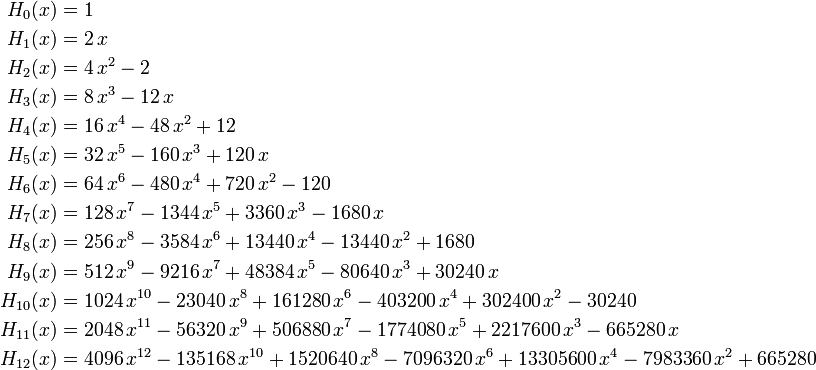\begin{align} H_0(x) &= 1 \\ H_1(x) &= 2\,x \\ H_2(x) &= 4\,x^2-2 \\ H_3(x) &= 8\,x^3-12\,x \\ H_4(x) &= 16\,x^4-48\,x^2+12 \\ H_5(x) &= 32\,x^5-160\,x^3+120\,x\\ H_6(x) &= 64\,x^6-480\,x^4+720\,x^2-120\\ H_7(x) &= 128\,x^7-1344\,x^5+3360\,x^3-1680\,x\\ H_8(x) &= 256\,x^8-3584\,x^6+13440\,x^4-13440\,x^2+1680\\ H_9(x) &= 512\,x^9-9216\,x^7+48384\,x^5-80640\,x^3+30240\,x\\ H_{10}(x) &= 1024\,x^{10}-23040\,x^8+161280\,x^6-403200\,x^4+302400\,x^2-30240 \\ H_{11}(x) &= 2048\,x^{11}-56320\,x^9+506880\,x^7-1774080\,x^5+2217600\,x^3-665280\,x \\ H_{12}(x) &= 4096\,x^{12}-135168\,x^{10}+1520640\,x^8-7096320\,x^6+13305600\,x^4-7983360\,x^2+665280 \end{align}